## 案例1-人脸识别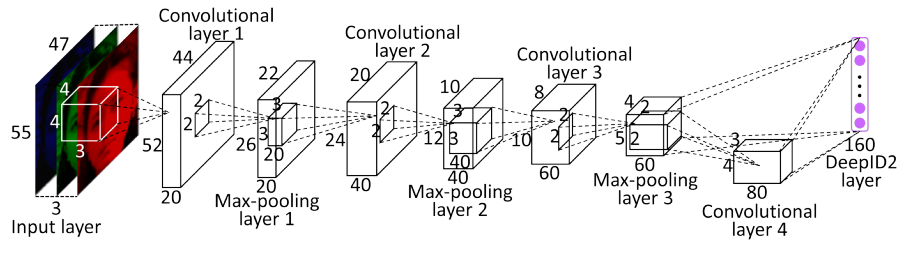DeepID2中共有两个损失函数,分别为人脸分类损失函数,对应于Caffe中的SoftmaxLoss:

Ident(f,t,θid)=pilogp^i=p^t

Ident(f,t,θid)$Ident(f,t,\theta_{id})$表示分类任务的损失函数，f$f$为DeepID2特征向量，θid$\theta_{id}$为Softmax层参数，pi$p_i$为属于第i$i$类的先验概率，即对于所有类别i$i$pi=0$p_i=0$，除了pt=1$p_t=1$t$t$为目标类别。

Verif(fi,fj,yij,θve)={12fifj2,12max(0,mfifj)2,if yij=1if yij=1

Verif(fi,fj,yij,θve)$Verif(f_i,f_j,y_{ij},\theta_{ve})$为度量学习任务的损失函数，fi,fj$f_i,f_j$为图片对（i,j$i,j$）的特征向量。yij=1$y_{ij}=1$表示（i,j$i,j$）属于同一类。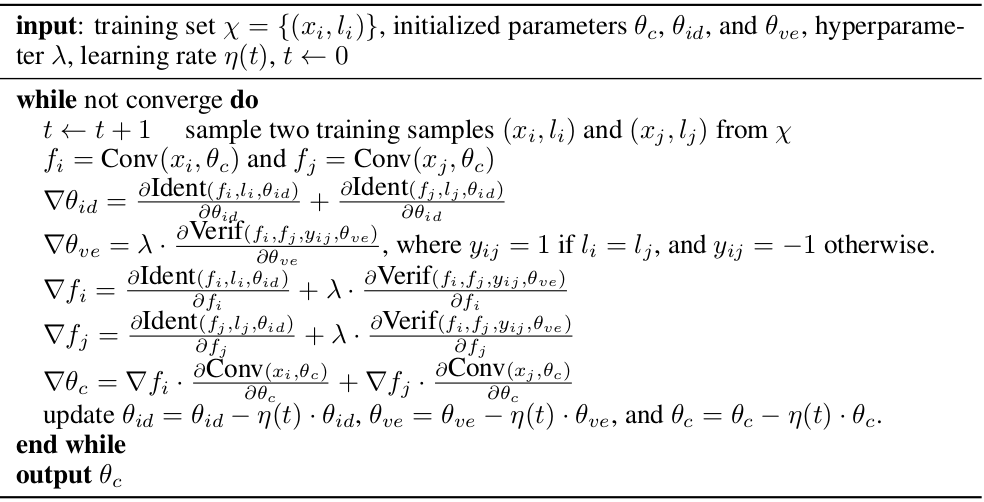“The face identification task increases the inter-personal variations by drawing DeepID2 extracted from different identities apart, while the face verification task reduces the intra-personal variations by pulling DeepID2 extracted from the same identity together”

• 分类任务旨在区分不同类，理论上，只要类别不同学习到的特征肯定不同，但是如果类别相同的话就无法保证特征一定相同，可能会出现同一个人脸由于表情不同学习到了不同的特征。
• 验证这个任务从另一个方面提取特征：只要类别相同（即相似），提取到的特征相似，类别不同，特征就不同。但是呢提取到的特征不能用于分类，只能做区分。所以说两个任务具有一定的互补性。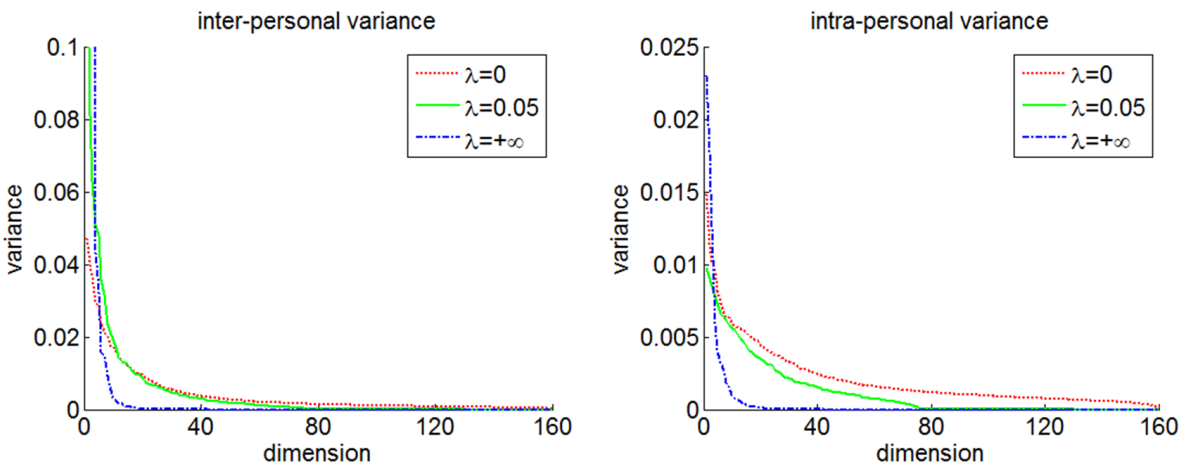λ$\lambda$是两个任务的比重，λ=0$\lambda=0$表示只用了分类任务，λ=+$\lambda=+\infty$表示只用了验证任务，λ=0.05$\lambda=0.05$时验证精度最高（即验证任务的比重为5%）。可以看见λ=0$\lambda=0$时类间距离最大，同时类内距离同样也很大。λ=+$\lambda=+\infty$时无论类间距离还是类内距离都很小，λ=0.05$\lambda=0.05$则做了一个很好的权衡。

## 案例2-Text-CNN

《Text-Attentional Convolutional Neural Network for Scene Text Detection》一文中介绍了TextCNN在传统CNN基础上加上了两个辅任务（auxiliary tasks）。

• 主任务：判断图片块是否含有字符（text/non-text，二分类问题）
• 辅任务1：给图片块中的字符分类（‘0-9，a-z，A-Z’，62类分类问题）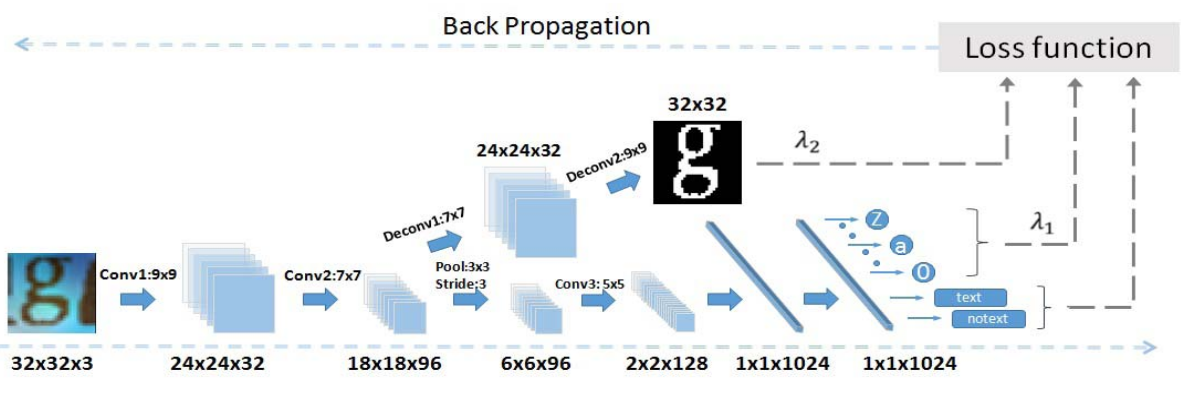argminWm,Wai=1NLm(ymi,f(xi;Wm))+i=1NaAλaLa(ya,f(xi;Wa))

LB(ybi,f(xi;Wb)=ybilog(p(ybi|xi;Wb)),

LL(yli,f(xi;Wl)=ylilog(p(yli|xi;Wl)),

LR(yri,f(xi;Wr)=yrif(xi;Wr)2.

## 案例3-细粒度车辆分类

《Embedding Label Structures for Fine-Grained Feature Representation》介绍了将SoftmaxLoss和TripletLoss结合在一个网络中进行多任务训练的方法。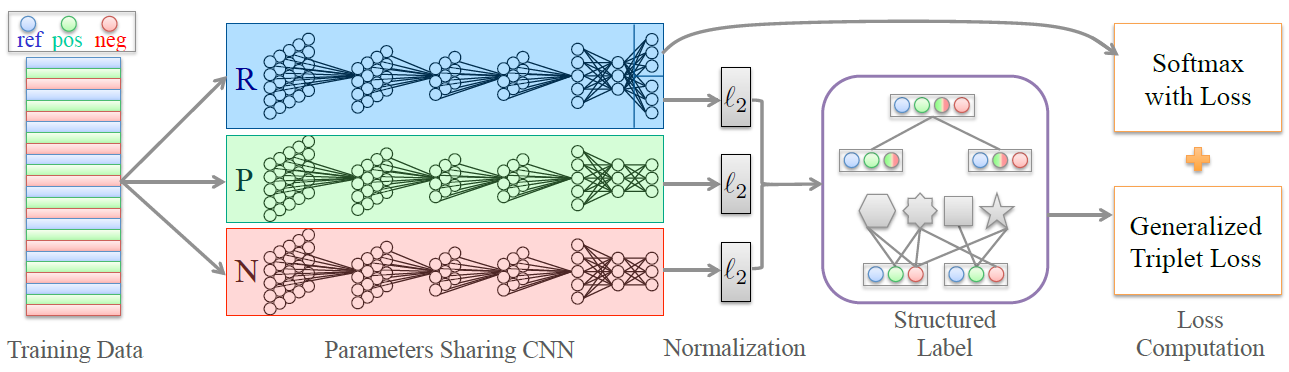SoftmaxLoss：

Es(r;l)=1Ni=1Nlogefs(ri,li)Cc=1efs(ri,c)

TripletLoss:

Et(r,p,n,m)=12Ni=1Nmax{0,D(ri,pi)D(ri,ni)+m}

E=λsEs(r;l)+(1λs)Et(r;p;n;m)